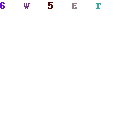A T-Square fractal is a relatively simple affair.T-Square

The procedure to create a T-Square starts off with a square canvas on which we are going to draw. It will work with rectangular canvases as well, but then the results will look slightly different.

When we call the Generate function, we need to pass to it the coordinates of the first square, which, to get the best fit into our canvas, we calculate the lengths of the sides of the square to be half the canvas sides, and then centre the square, essentially making the top left corner a quarter of the way to the right and down of the origin.

The first thing we do is draw a solid square using our coordinates.

Now, until we reach our desired recursion depth, we generate four new squares, which have half the width and height, and make the centres of each of these squares to be centred on each of the four corners of the original square.

This process is then repeated until we have recursed far enough.

```        public void GenerateTSquare(Graphics g, int iIterations, double iLeft, double iTop, double iWidth, double iHeight, Color oColor)
{
g.FillRectangle(new SolidBrush(oColor), (float)iLeft, (float)iTop, (float)iWidth, (float)iHeight);
if (iIterations > 1)
{
double dNewWidth = iWidth / 2.0;
double dNewHeight = iHeight / 2.0;

GenerateTSquare(g, iIterations - 1, iLeft - (dNewWidth / 2.0), iTop - (dNewHeight / 2.0), dNewWidth, dNewHeight, oColor);
GenerateTSquare(g, iIterations - 1, iLeft + iWidth - (dNewWidth / 2.0), iTop - (dNewHeight / 2.0), dNewWidth, dNewHeight, oColor);
GenerateTSquare(g, iIterations - 1, iLeft - (dNewWidth / 2.0), iTop + iHeight - (dNewHeight / 2.0), dNewWidth, dNewHeight, oColor);
GenerateTSquare(g, iIterations - 1, iLeft + iWidth - (dNewWidth / 2.0), iTop + iHeight - (dNewHeight / 2.0), dNewWidth, dNewHeight, oColor);

}
}

```